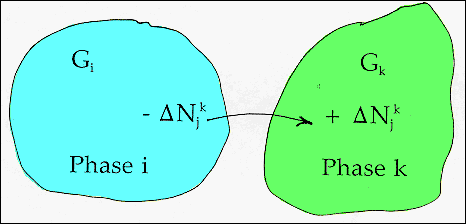Phase Diagrams The Gibbs Phase Rule · Two phases, phase i and phase k, are illustrated. Each phase contains a number of different atom types, i, j, ..... · Let: * Nij = Number of moles of j-atoms in phase i. * Nkj= Number of moles of j-atoms in phase k.* Gi , Gk are the Gibbs functions of the phases i and k respectively. · Consider a process which transfers j-atoms from phase i to phase k. In this process: - ΔNij = +ΔNkj as the total number of atoms in the system are conserved.